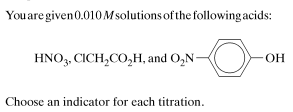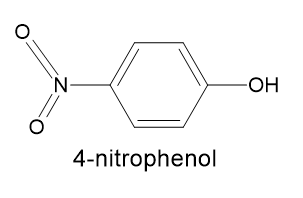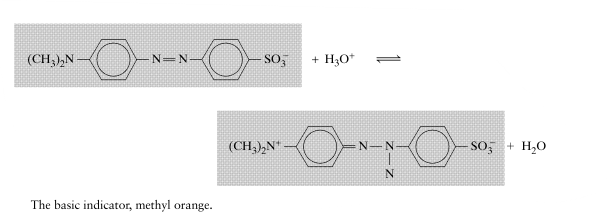# Indicators for Acids Titration

## Recommended Posts

Posted (edited)How to choose indiacator here for these above mentioned Acids titation?

Is the below-given  chemical compound structure of 4- nitrophenol same as given in the above question?Edited by Dhamnekar Win,odd
##### Share on other sites

11 minutes ago, Dhamnekar Win,odd said:

How to choose indiacator here for these above mentioned Acids titation?

Is the below-given  chemical compound structure of 4- nitrophenol same as given in the above question?

What do you think about the relative tendency to release H+, for these 3 molecules?

##### Share on other sites

45 minutes ago, Dhamnekar Win,odd said:

How to choose indiacator here for these above mentioned Acids titation?

Is the below-given  chemical compound structure of 4- nitrophenol same as given in the above question?

Yes it is

##### Share on other sites

6 hours ago, Dhamnekar Win,odd said:

How to choose indiacator here for these above mentioned Acids titation?

Is the below-given  chemical compound structure of 4- nitrophenol same as given in the above question?

The structure you have drawn for 4-nitrophenol is not quite right. You can't show 5 full bonds from nitrogen, as the n=2 valence shell has only 4 orbitals in it (s + 3x p.)

It is often shown as as a resonance hybrid of a pair of structures with a double bond to one O atom and a single dative bond to the other. This gives the 2 O atoms a partial net -ve charge and the N atom a +ve charge, which is what gives the nitro group its electron-withdrawing properties on the benzene ring.

Sometimes it is drawn as a mix of the 2 structures, with a single solid line to each O atom plus a dotted line to each, giving a bond order of 1 1/2 to each, for example in this Wiki article: https://en.wikipedia.org/wiki/Nitro_compound. The polarity of the nitro group, resulting from the dative bond, is crucial to understanding its influence.

##### Share on other sites

The following question is related to original question to some extent.  Hence I am asking here.☺️

What is the explanation for the following acid-base behavior of Methyl Orange, an Indicator?I am working on the original question. Any Chemistry help will be accepted.

##### Share on other sites

Posted (edited)

The drawing on the right molecule is wrong. We have here mesomerie behaviour, the aromatic System is broken  in the left Ring.

Edited by chenbeier
##### Share on other sites

Posted (edited)

1)In the titration of 0.010 M HNO3  (Nitric acid), the reaction equation is NaOH + HNO3  → NaNO3 + H2O. At the equivalence point, [NaNO3] = 0.005 M. At the equivalence point, equal volume of  hydroxide solution have been  added to the acid solution and all  of the hydronium ions resulting from HNO3 have  been  converted to water. The solution at this point is simply an  aqueous solution of sodium nitrate.  Na + OH-  + H3O+  + (NO3)-   ⇌ 2H2O + Na + (NO3)-.

Since neither sodium ions nor nitrate ions reacts with water, the solution is neither acidic nor basic; that is [H3O+] = [OH-] = 10-7 M. pH at the equivalence point is therefore 7.0  Indicator such as Phenol red (C19H14O5S) must be used which can respond to a pH of 7, the exact pH at the equivalence point.

2) In the titration of 0.010 M of ClCH2COOH (Chloroacetic acid), the reaction equation is ClCH2CO2-  + H2O  →  ClCH2CO2H  + OH-

x = [OH-] = [ClCH2CO2H] ;  0.0050 - x = [ ClCH2COO-];

Kb = $\frac {x^2}{0.0050 - x}= \frac {K_w}{K_a}= \frac {10^{-14}}{1.4 \times 10^{-3}}= 7.1 \times 10 ^{-12}$

x= 1.9 × 10-7 M = [OH-]; pOH = -log(1.9 × 10-7 ) =6.72 ; pH =7.28.

Indicator such as Phenol red (C19H14O5S) must be used which can respond to a pH of 7.28, the exact pH at the equivalence point.

3)  In the titration of 0.010 M of O2NC6H4OH (o-nitrophenol), the reaction equation is O2NC6H4O- + H2O → O2NC6H4OH + OH-.

x= [OH-] = [ O2NC6H4OH] and 0.0050 - x = [O2NC6H4O-];

Kb = $\frac{x^2}{0.0050 - x}= \frac{K_w}{K_a}= \frac{10^{-14}}{6.8 \times 10^{-8}} = 1.4 \times 10^{-7}$

x = 2.6 × 10-5  M ; pOH = -log (2.6 × 10-5 ) = 4.58; pH = 9.42

Indiactor such as Phenolphthalein must be used

Edited by Dhamnekar Win,odd

## Create an account

Register a new account